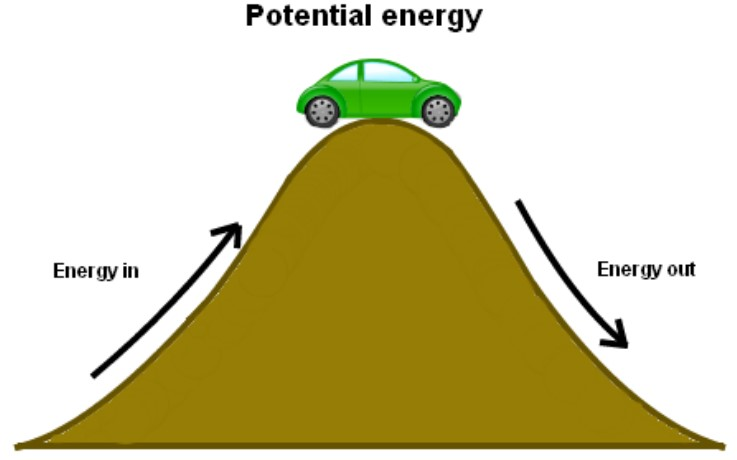# Gravitational Potential Energy Calculator

To find potential energy, enter the values of mass, acceleration due to gravity, and height of the object in the potential energy calculator.

kg
m/s2
m

Give Us Feedback

Potential energy calculator aka gravitational potential energy calculator can find the value of potential energy if the values of the mass, height, and gravitational acceleration are given.

## What is Gravitational Potential energy?Gravitational potential energy is the energy stored in an object as the result of its vertical position or height.

The name gravitational is given due to the effect of gravity on the object’s potential energy. The SI unit of potential energy is Joules.

Need to calculate kinetic enery? Use our kinetic energy calcuator.

## Potential energy formula

Formula or equation for gravitational potential energy is:

G.P.E =m×g×h

In this equation:

m is mass,

g is the value of gravitation, and

h is the height.

## How to calculate potential energy?

Example:

What will be the gravitational potential energy of a ball if its mass is 2 kg and it is placed on a cliff having height of 10 meters?

Solution

Step 1: Identify the values.

m = 2 kg

h = 10 m

g = 9.8 ms-2

Step 2: Put the values in the formula mentioned above.

G.P.E = mgh

G.P.E = 2×9.8×10

G.P.E  = 196 j

### Math Tools